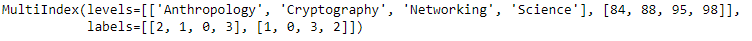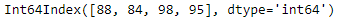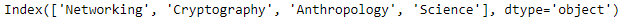# Python | Pandas MultiIndex.droplevel()

• Last Updated : 24 Dec, 2018

Python is a great language for doing data analysis, primarily because of the fantastic ecosystem of data-centric python packages. Pandas is one of those packages and makes importing and analyzing data much easier.

Pandas` MultiIndex.droplevel()` function return Index with requested level removed. If MultiIndex has only 2 levels, the result will be of Index type not MultiIndex..

Syntax: MultiIndex.droplevel(level=0)

Parameters :
level : int/level name or list thereof

Returns : index : Index or MultiIndex

Example #1: Use `MultiIndex.droplevel()` function to drop the 0th level of the MultiIndex.

 `# importing pandas as pd``import` `pandas as pd`` ` `# Create the MultiIndex``midx ``=` `pd.MultiIndex.from_arrays([[``'Networking'``, ``'Cryptography'``, ``                                     ``'Anthropology'``, ``'Science'``], ``                                             ``[``88``, ``84``, ``98``, ``95``]])`` ` `# Print the MultiIndex``print``(midx)`

Output :Now let’s drop the 0th level of the MultiIndex.

 `# drop the 0th level.``midx.droplevel(level ``=` `0``)`

Output :As we can see in the output, the function has dropped the 0th level and returned an Index object.

Example #2: Use `MultiIndex.droplevel()` function to drop the 1st level of the MultiIndex.

 `# importing pandas as pd``import` `pandas as pd`` ` `# Create the MultiIndex``midx ``=` `pd.MultiIndex.from_arrays([[``'Networking'``, ``'Cryptography'``,``                                     ``'Anthropology'``, ``'Science'``],``                                              ``[``88``, ``84``, ``98``, ``95``]])`` ` `# Print the MultiIndex``print``(midx)`

Output :Now let’s drop the 1st level of the MultiIndex.

 `# drop the 1st level.``midx.droplevel(level ``=` `1``)`

Output :As we can see in the output, the function has dropped the 1st level and returned an Index object.

My Personal Notes arrow_drop_up